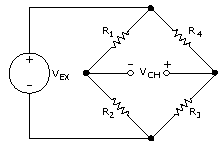How to connect a PT1000 to an Arduino based PLC
PT100 for industrial automation with an Arduino PLC controller
10 diciembre, 2018 porAlejandro Jabalquinto

## Introduction

This post, it is showed how to connect a Pt1000 (thermally sensitive resistor or RTD resistance temperature detector) to an Arduino based PLC for industrial control and automation solutions.

• # Industrial Shields boards:

## Description

The first thing that we have to know is what our temperature range of operation is. Also in this temperature range, we need to know the range value of the thermistor. For example, if our range temperature operation is between -20ºC and 100ºC, the range thermistor value would be between 922 ohms and 1385 ohms.

So, the main idea is to adapt this changeable resistor to a 0-10V. How to do that?

There are many ways to adapt the signal. The simple one just used a tension devisor to transform the resistive value of the Pt1000 to a quantitative value.

For example, connecting a resistor of 1Kohm between a power supply of 12V and also connecting the thermistor between this input and GND. Therefore, with this configuration, the input value on -20ºC would be 5,75V and on 100ºC would be on 6,97V. So our voltage range it’s between [5,75V – 6,97V]. We just have a 1,21 difference voltage between our range. The main question with this method is: Have we enough resolution and accuracy? This just depends on the application.

The Arduino boards have an ADC of 10 bits. This is a range of 1024. So with this range, we have a resolution of 0,0097V [on 0V-10V]. This means that in our case, we will have a °C resolution of:

10/1024 = 0,0097V (total voltage resolution)

1,21/0,0097V = 124 (number of different values that we can measure on our example, this means that we will just use 124 range instead of 1024 that offers the ADC)

120ºC (range)/ 124 = 0,96ºC (°C resolution)

This is why this installation is really simple but not enough reliable. We have to decide if we can implement that or find another way to adapt the thermistor.

Next is shown one of the methods to increase this work voltage range.

We can use a Wheatstone bridge.Wheatstone's bridge has an advantage, this advantage is that you can calculate the values of the resistor (R1 and R4, normally R2 is a potentiometer to correct the resistors' error) to have a 0V value between Vch (see on the image) for our -20ºC (922 ohms). We can calculate this value from these formulas:

R3 (thermistor) = R3 · R2 / R1

By doing that, we will be able to get the value of 0V between Vch – and Vch+. After that, we just have to calculate the value of Vch when the value of the resistor will be 1358 ohms. Normally this value is around several mV. So the final part of thermistor adaption is to amplify this signal [0- several mV] to [0 – 10V]. With a good differential OPAMP it is able to do it.

A differential amplifier made from an op-amp and four resistors is perfectly fine when (and only when) the input sources are much, much lower impedance than the resistors used. So, we shall use a differential amplify like this:

On the market, there are also some devices that allow to combers what is explained in this post. This is also a good option and completely reliable.Alejandro Jabalquinto
10 diciembre, 2018

## ¿Buscas tu controlador lógico programable ideal?

### ¿Quieres más información?

¡Rellena el formulario!